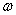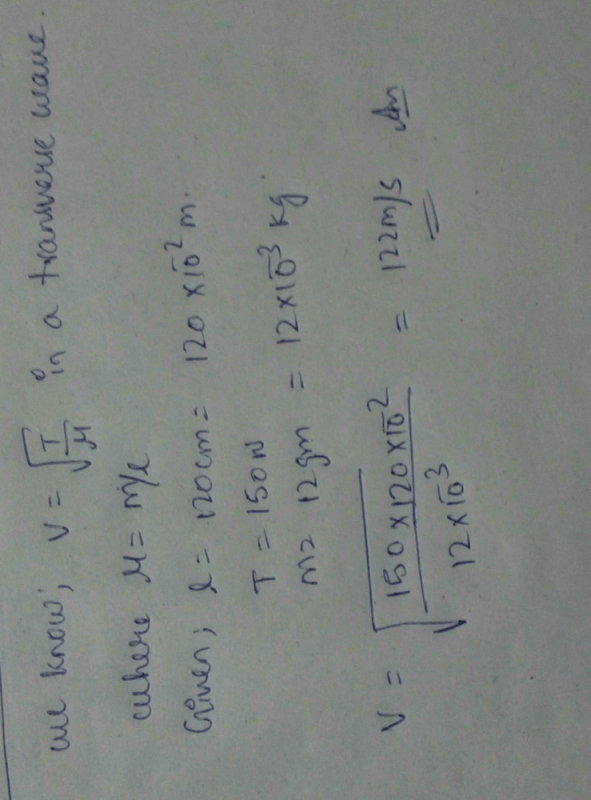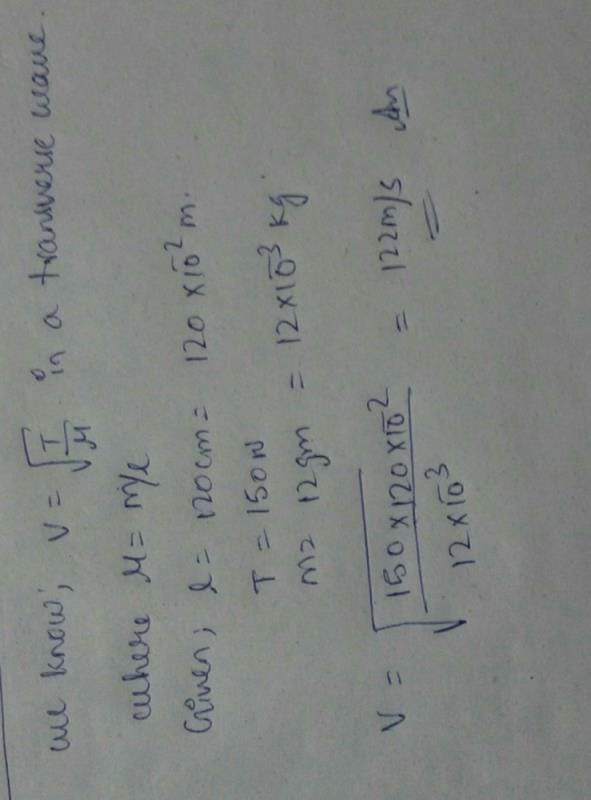NEET  >  Test: Progressive Waves

# Test: Progressive Waves

Test Description

## 10 Questions MCQ Test Physics Class 11 | Test: Progressive Waves

Test: Progressive Waves for NEET 2023 is part of Physics Class 11 preparation. The Test: Progressive Waves questions and answers have been prepared according to the NEET exam syllabus.The Test: Progressive Waves MCQs are made for NEET 2023 Exam. Find important definitions, questions, notes, meanings, examples, exercises, MCQs and online tests for Test: Progressive Waves below.
Solutions of Test: Progressive Waves questions in English are available as part of our Physics Class 11 for NEET & Test: Progressive Waves solutions in Hindi for Physics Class 11 course. Download more important topics, notes, lectures and mock test series for NEET Exam by signing up for free. Attempt Test: Progressive Waves | 10 questions in 10 minutes | Mock test for NEET preparation | Free important questions MCQ to study Physics Class 11 for NEET Exam | Download free PDF with solutions
 1 Crore+ students have signed up on EduRev. Have you?
Test: Progressive Waves - Question 1

### Waves associated with moving protons, electrons, neutrons, atoms are known as

Detailed Solution for Test: Progressive Waves - Question 1

Matter waves are a central part of the theory of quantum mechanics, being an example of wave–particle duality. All matter exhibits wave-like behavior. For example, a beam of electrons can be diffracted just like a beam of light or a water wave.
Matter is made of atoms, and atoms are made protons, neutrons and electrons. These are not macroscopic particles.

Test: Progressive Waves - Question 2

### Maximum destructive inference between two waves occurs when the waves are out of the phase by

Detailed Solution for Test: Progressive Waves - Question 2

Let the waves be
y1 = Asin(wt)
y2 = Asin(wt + φ)
If φ = π the interference is destructive.
Destructive interference occurs when the maxima of two waves are 180 degrees out of phase: a positive displacement of one wave is cancelled exactly by a negative displacement of the other wave. The amplitude of the resulting wave is zero. The dark regions occur whenever the waves destructively interfere.
Hence B is the correct answer.

Test: Progressive Waves - Question 3

### In what types of waves can we find capillary waves and gravity waves?

Detailed Solution for Test: Progressive Waves - Question 3

A longer wavelength on a fluid interface will result in gravity–capillary waves which are influenced by both the effects of surface tension and gravity, as well as by fluid inertia. On the open ocean, much larger ocean surface waves (seas and swells) may result from coalescence of smaller wind-caused ripple-waves.

Test: Progressive Waves - Question 4

To the nearest order of magnitude, how many times greater than the speed of sound is the speed of light?

Detailed Solution for Test: Progressive Waves - Question 4

Speed of sound in air is 343 m/s or we can say approx 300m/s
And speed of light is approx 300,000,000 m/s
Clearly the ratio is 106

Test: Progressive Waves - Question 5

The angular frequencyis related to the time period T by

Detailed Solution for Test: Progressive Waves - Question 5

Angular frequency is associated with the number of revolutions an object performs in a certain unit of time. In that sense is related to frequency but in terms of how many times it turns a full period of motion in radians units.
The formula of angular frequency is given by:
Angular frequency = 2 π / (period of oscillation)
ω = 2π / T = 2πf
Where we have:
ω: angular frequency
T: period
f: frequency
Hence C is correct.

Test: Progressive Waves - Question 6

A steel wire 120 cm long has mass 12 g attached to it. If the wire is under a tension of 150N, what is the speed of transverse wave in the wire?

Detailed Solution for Test: Progressive Waves - Question 6Test: Progressive Waves - Question 7

In a wave which quantity is transferred from one point to the other.

Detailed Solution for Test: Progressive Waves - Question 7

'Wave' is a common term for a number of different ways in which energy is transferred: In electromagnetic waves, energy is transferred through vibrations of electric and magnetic fields. In sound waves, energy is transferred through vibration of air particles or particles of a solid through which the sound travels.

Test: Progressive Waves - Question 8

What is the range of frequency of audible sound?

Detailed Solution for Test: Progressive Waves - Question 8

20 to 20,000 Hz
The SI unit of audio frequency is the hertz (Hz). It is the property of sound that most determines pitch. The generally accepted standard range of audible frequencies for humans is 20 to 20,000 Hz, although the range of frequencies individuals hear is greatly influenced by environmental factors.

Test: Progressive Waves - Question 9

In a transverse wave, the constituents of the medium oscillate ___________to the direction of wave propagation and in a longitudinal wave they oscillate ________to the direction of propagation.

Detailed Solution for Test: Progressive Waves - Question 9

Both transverse and longitudinal waves are mechanical waves where they need material medium to propagate. The motion of transverse wave is perpendicular to the direction of the wave motion, and parallel for longitudinal waves.

Test: Progressive Waves - Question 10

The ratio of velocity of sound in hydrogen and oxygen at STP is __________

Detailed Solution for Test: Progressive Waves - Question 10

vH/vO = √(MO/MH)
=√(32/2)=4:1.

## Physics Class 11

127 videos|464 docs|210 tests
 Use Code STAYHOME200 and get INR 200 additional OFF Use Coupon Code
Information about Test: Progressive Waves Page
In this test you can find the Exam questions for Test: Progressive Waves solved & explained in the simplest way possible. Besides giving Questions and answers for Test: Progressive Waves, EduRev gives you an ample number of Online tests for practice

## Physics Class 11

127 videos|464 docs|210 tests

### How to Prepare for NEET

Read our guide to prepare for NEET which is created by Toppers & the best Teachers